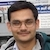# NAEST 2017 Screening Test: Phase DifferenceBy

Consider a rigid body in Simple Harmonic Motion (SHM) of time period T. Each point on the body is in phase i.e., the phase difference between any two points on this body is zero. Now, consider another rigid body in SHMs of same time period T. The phase difference between a point on the first body and a point on the second point remains constant with time.

Question 1: Assume small oscillations of the toy so that displacement of a small portion can be treated as on a straight line. Call a portion of head just above the neck as A, the portion of the body just below the neck as B, the end of the right arm as C and the end of the left arm as D. The phase difference between oscillation of A and B is

1. 0 deg
2. 90 deg
3. 180 deg
4. 270 deg

Question 2: The phase difference between oscillation of C and D is

1. 0 deg
2. 90 deg
3. 180 deg
4. 270 deg

Answer: Let us have a look at the construction of the toy. It has three parts

1. head where point A is located
2. trunk with point C on left, point B in middle and point D on right and
3. base (white color).
The trunk is connected with a rod. This rod makes small oscillations about an axis passing through some point in the base and normal to the plane formed by A, B and C. The displacement of C and D are out of phase i.e., phase difference between these points is 180 deg (try to write the equation for the displacement of C and D).

The head and trunk are joined together by a joint. The head is free to rotate about an axis passing through this joint and perpendicular to a plane formed by B, C, and D. The points A and B are on two different bodies. These points oscillate about an axis perpendicular to the line joining A and B and passing through the joint. The displacement of A and B are out of phase i.e., the phase difference between these points is 180 deg (try to write the equation for the displacement of A and B).